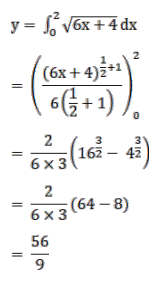# Mark against the correct answer in the following:Question:

Mark $(\sqrt{ })$ against the correct answer in the following:

$\int_{0}^{2} \sqrt{6 x+4} d x=?$

A. $\frac{64}{9}$

B. 7

C. $\frac{56}{9}$

D. $\frac{60}{9}$

Solution: